# Convert Bytes to MB or GB in Excel – 3 Methods!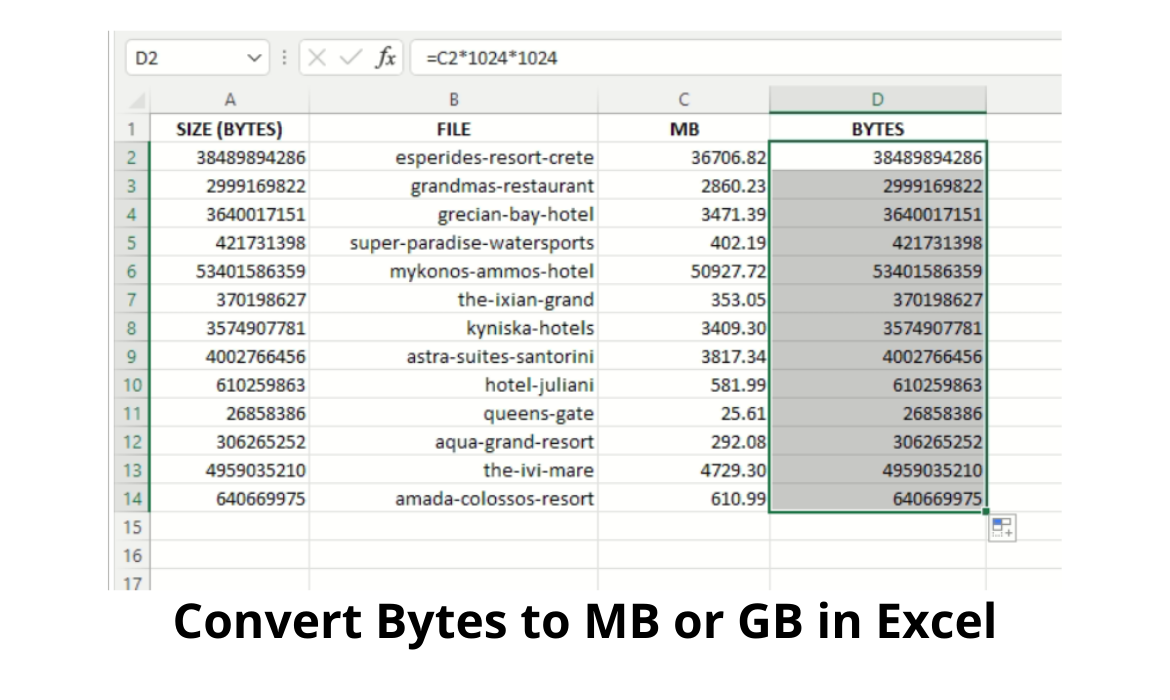In the metric system, units of measure use prefixes like kilo and mega to depict orders of magnitude.

This makes referring to and comparing large values or quantities, much easier.

Since the metric system uses base 10, the orders of magnitude are 10 to some power, usually one that is divisible by 3, such as 103 for kilo, 106 for mega, 109 for giga, and so on.

So for example, the unit of measure of length is the meter.

One thousand meters, which can also be written as 1 x 103 meters, is typically referred to as 1 kilometer.

One million meters can be written as 1 x 106 meters and though rare, can be referred to as 1 megameter.

One million meters can also be written as 1,000 x 103 meters.

This is in fact more common and is referred to as one thousand kilometers.

While you may see prefixes such as kilo, mega, and giga written in full, it is more common to see them in their abbreviated form of k, M, and G respectively (e.g. 100 kilometers would be more commonly written as 100 km).

The same principle is applied to other units of measure, such as frequency, where the unit of measure is the Hertz, and electrical power, where the unit of measure is the Watt (W).

We therefore find kW (kilowatts), MW (megawatts), GW (gigawatts) and even TW (terawatts) in common use.

So it is not surprising that the same principle is used when referring to the capacity of computer memory.

This not only applies to computer RAM (and ROM), but also disk space, file sizes, network transfer rates, and generally anything that refers to capacity or throughput (think Internet connection speed).

There is however, one slight difference. As was stated previously, the metric system uses base 10, whereas computers use base 2, also referred to as binary.

One kilobyte or 1 kB, is not exactly 1,000 bytes, but is in actuality 1024 bytes.

This is because we cannot raise 2 to an integer power to get exactly 1000.

The closest is 2 to the power of 10 (210) which is exactly 1,024.

Hence, 1 kB is equal to 1,024 bytes. One megabyte, or 1 MB, is 1024 x 1024 bytes which is 1,048,576 bytes.

Similarly, 1 GB is 1,073,741,824 bytes.

But as we can see, it is much easier to refer to (and remember) 1 GB, than a long, ten digit number.

A side note before proceeding further. Since we are dealing with binary systems and dividing and multiplying by 1024 (or 210) to perform any conversions, the correct prefixes are kiB, MiB, GiB and TiB, as opposed to kB, MB, GB and TB (i.e. without the i), used in the metric system.

So how does all this fit in with Excel?

## Convert Bytes to MB or GB in Excel

Nowadays, it’s not uncommon to have to refer to memory capacity, be it in a sheet containing various computer configurations, file sizes, or components in a hardware stock list.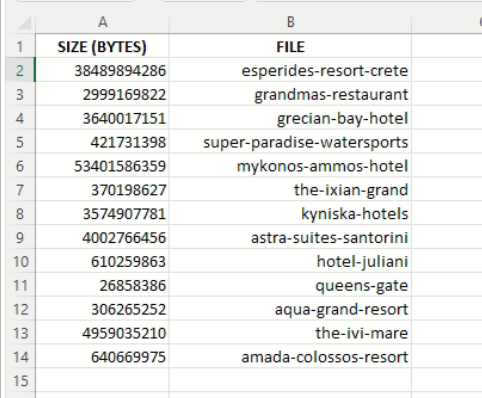The above example sheet, contains a short list of filenames and their respective sizes in bytes. But we want to convert the file sizes to megabytes and gigabytes.

We will do this using a few different methods.

Since the file sizes in the sheet are in bytes, dividing the sizes by 1024 will give us the equivalent size in kilobytes.

We need to divide by a further 1024 to get the size in megabytes. And then one more time, to get the size in gigabytes.

Since conversion involves the simple mathematical operation of division, we can simply do this with a formula using division.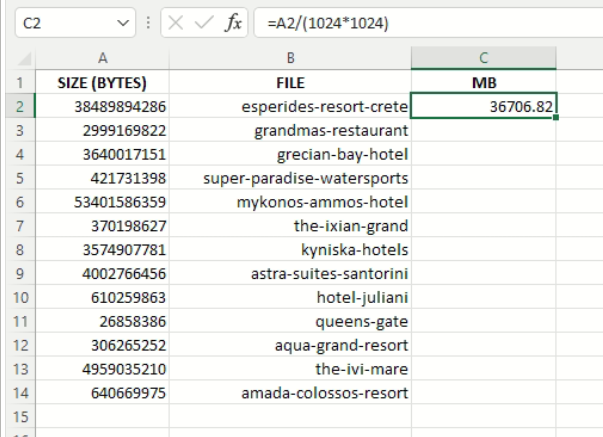With reference to the size of the first file, our formula in cell C2 needs to divide the contents of cell A2 by (1024 x 1024) in order to show the size in megabytes.

We could also divide by 1,048,576, which is the result of multiplying 1024 by 1024, but it is easier to just work with multiples of 1024.

It is also easy to see whether we are converting to kilobytes, megabytes, or gigabytes, by counting how many times we have divided by 1024 in the formula. In this case two times, so we are converting to megabytes.

## Formula to Convert to Megabytes

So, to convert to megabytes, the formula required is

=A2/(1024*1024)

The result is as shown in cell C2 in the snapshot above, namely, 36706.82 MB.

The two places after the decimal point are optional, and depend on what formatting has been applied.

You can change this by right mouse clicking inside the cell, and from the context menu select Format Cells…

The Format Cells dialog box will then open that allows you to alter the format, including the number of decimal places.

We can now propagate our formula to the remaining cells in column C, by grabbing the fill handle in the bottom right hand corner of cell’s C2 bounding box, and dragging it down to cell C14.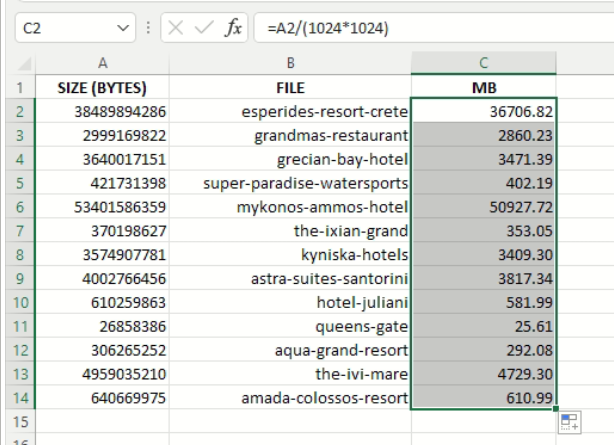The result is that column C now displays the sizes in megabytes.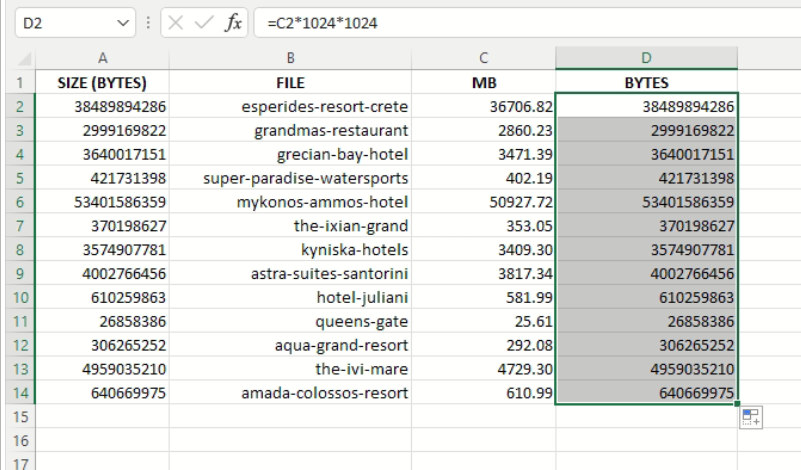To test that this also works converting other way (i.e. we can convert from megabytes to bytes), all we need to do to our formula is to multiply rather than divide.

The formula is shown in the formula bar in the snapshot above,

=C2*1024*1024

where we take the megabyte value in C2 and convert it back to bytes in D2.

Column D displays the converted sizes and matches exactly the sizes shown in column A, the original sizes of the files.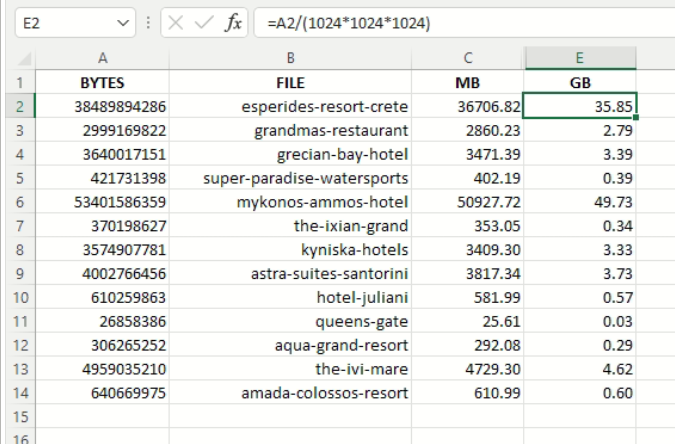Finally, we show how to convert to gigabytes in column E in the snapshot above, using the formula

=A2/(1024*1024*1024)

All the above formulas are simple mathematical equations. Since 1024 can also be written as 210, we can change the formula so that it divides by 210 for kilobytes, 220 for megabytes, or 230 for gigabytes.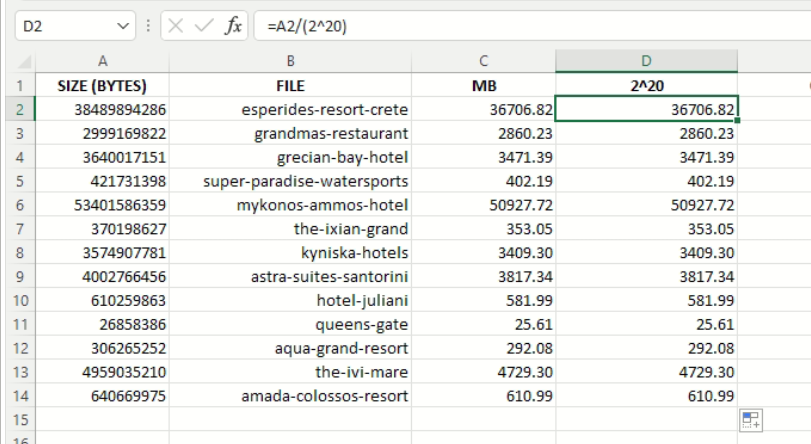Column D in the snapshot above uses the new formula

=A2/(2^20)

to give us the size in megabytes. It is more succinct and gives exactly the same results as column C, proving that the two formulas are identical.

2^20 is how to write two to the power of twenty in Excel, and is equivalent to 1024 x 1024.

2^30 would be equivalent to 1024 x 1024 x 1024, and would give the size in gigabytes.

Excel being what it is, there is yet another way to express a number raised to a power.

It is with the built in function, POWER. The definition is given below.

## POWER function

Returns the result of a number raised to a power.

## Syntax

POWER(number, power)

The POWER function syntax has the following arguments:

• Number    Required. The base number. It can be any real number.
• Power    Required. The exponent to which the base number is raised.

We can now rewrite the formula

=A2/(2^20)

as

=A2/POWER(2,20)

Which form is best is a matter of personal preference.

Now if you thought that was simple, it gets even easier.

Excel has a function that automatically performs the conversion for you.

The function is CONVERT and the definition is given below.

## CONVERT

C

Converts a number from one measurement system to another. For example, CONVERT can translate a table of distances in miles to a table of distances in kilometers.

## Syntax

CONVERT(number,from_unit,to_unit)

Number     is the value in from_units to convert.

From_unit     is the units for number.

To_unit     is the units for the result. CONVERT accepts the following text values (in quotation marks) for from_unit and to_unit.

### Notes

• The following list outlines the available unit conversions by category:
• Weight – u, grain, g, ozm, lbm, stone, sg, cwt, uk_cwt, ton, uk_ton
• Distance – ang, Picapt, pica, in, ft, yd, m, ell, mi, survey_mi Nmi, ly, parsec
• Time – sec, min, hr, day, yr
• Pressure – Pa, mmHg, Torr, psi, atm
• Force – dyn, pond, N, lbf
• Energy – eV, e, J, flb, c, cal, BTU, Wh, HPh
• Power – W, PS, HP
• Magnetism – ga, T
• Temperature – C, F, K, Rank, Reau
• Volume – ang^3, Picapt^3, tsp, tspm, tbs, in^3, oz, cup, pt, uk_pt, qt, l, uk_qt, gal, uk_gal, ft^3, bushel, barrel, yd^3, m^3, MTON, GRT, mi^3, Nmi^3, ly^3
• Area – ang^2, Picapt^2, in^2, ft^2, yd^2, m^2,ar, Morgen,uk_acre, us_acre, ha, mi^2, Nmi^2, ly^2
• Information – bit, byte
• Speed – m/hr, mph, kn, admkn, m/s
• If the input data types are incorrect, CONVERT returns the #VALUE! error value.
• If the unit does not exist, CONVERT returns the #N/A error value.
• If the unit does not support a binary prefix, CONVERT returns the #N/A error value.
• Unit names and prefixes are case-sensitive.
• If the units are in different groups, CONVERT returns the #N/A error value. For example, CONVERT(13.2,”ft”,”C”) would result in an error as a result of an attempted conversion from distance units to temperature units.

Note the statement in the last bullet point in the definition.

You cannot convert feet to Celsius.

CONVERT only converts within the same category of units.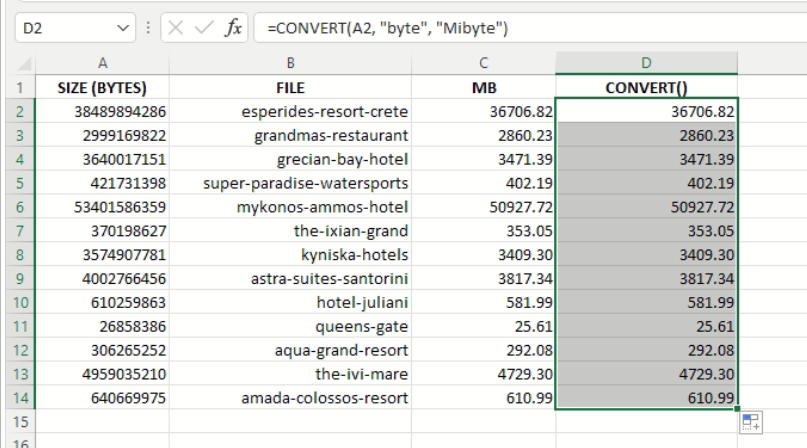We can now go ahead and create our new formula, this time using the CONVERT function, putting it in Cell D2.

The formula is

=CONVERT(A2, “byte”, “Mibyte”)

which we propagate to the other cells in column D.

This produces the exact same results as column C, which still has the original formula we used to convert to megabytes,

=A2/(1024*1024)

For those not used to or comfortable with dealing in bytes and megabytes, using the CONVERT function may be the easiest and most readable format.

Without a lot of thought, you can convert back and forth, and even to bits (8 bits equal one byte).

The table below lists the Excel Identifiers that must be used for the parameters start_unit and end_unit when referring to the units in the CONVERT function.

Note also, that the Excel Identifiers are case sensitive, and will cause a #N/A error if even one letter is not of the correct case.

Unit Excel Identifier
Byte byte
Kilobyte kibyte
Megabyte Mibyte
Gigabyte Gibyte
Terabyte Tibyte

## Conclusion

Converting bytes to kilobytes, megabytes, gigabytes, terabytes, and back, is not very difficult, requiring some simple mathematical equations.

Yet as we saw, there are quite a few different ways of achieving this with Excel, including the use of built in functions, and especially, the very convenient CONVERT function.

Which method is best, depends on personal preference, although for most people, the CONVERT function would be the quickest and easiest.

We hope this tutorial on how to convert bytes to MB or GB was useful and helps you achieve your goal!

Costas has a wide range of experience in Information Technology covering computer hardware, programming, telecommunications, networking, web services, and general IT support. He's worked in various roles such as PHP programmer and web developer, technical and desktop support, hardware repair, system administration. Costas has excellent background in Microsoft Windows and Office Suite (Excel, Word, Powerpoint, etc), as well as a thorough understanding of Networking and Hardware maintenance.